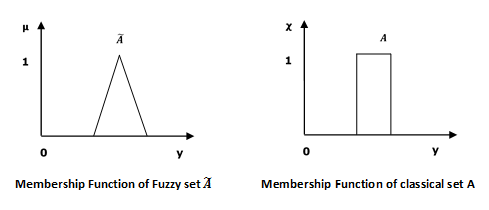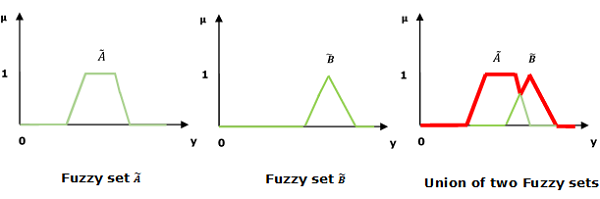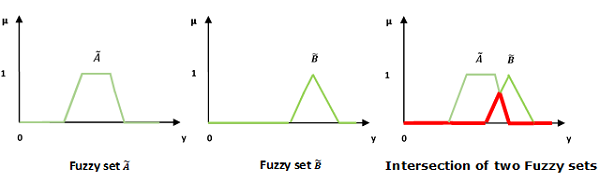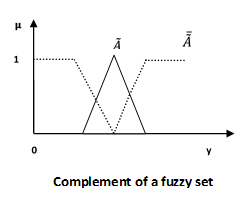# Fuzzy Logic - Set Theory

Fuzzy sets can be considered as an extension and gross oversimplification of classical sets. It can be best understood in the context of set membership. Basically it allows partial membership which means that it contain elements that have varying degrees of membership in the set. From this, we can understand the difference between classical set and fuzzy set. Classical set contains elements that satisfy precise properties of membership while fuzzy set contains elements that satisfy imprecise properties of membership.## Mathematical Concept

A fuzzy set $\widetilde{A}$ in the universe of information $U$ can be defined as a set of ordered pairs and it can be represented mathematically as −

$$\widetilde{A} = \left \{ \left ( y,\mu _{\widetilde{A}} \left ( y \right ) \right ) | y\in U\right \}$$

Here $\mu _{\widetilde{A}}\left ( y \right )$ = degree of membership of $y$ in \widetilde{A}, assumes values in the range from 0 to 1, i.e., $\mu _{\widetilde{A}}(y)\in \left [ 0,1 \right ]$.

## Representation of fuzzy set

Let us now consider two cases of universe of information and understand how a fuzzy set can be represented.

### Case 1

When universe of information $U$ is discrete and finite −

$$\widetilde{A} = \left \{ \frac{\mu _{\widetilde{A}}\left ( y_1 \right )}{y_1} +\frac{\mu _{\widetilde{A}}\left ( y_2 \right )}{y_2} +\frac{\mu _{\widetilde{A}}\left ( y_3 \right )}{y_3} +...\right \}$$

$= \left \{ \sum_{i=1}^{n}\frac{\mu _{\widetilde{A}}\left ( y_i \right )}{y_i} \right \}$

### Case 2

When universe of information $U$ is continuous and infinite −

$$\widetilde{A} = \left \{ \int \frac{\mu _{\widetilde{A}}\left ( y \right )}{y} \right \}$$

In the above representation, the summation symbol represents the collection of each element.

## Operations on Fuzzy Sets

Having two fuzzy sets $\widetilde{A}$ and $\widetilde{B}$, the universe of information $U$ and an element 𝑦 of the universe, the following relations express the union, intersection and complement operation on fuzzy sets.

### Union/Fuzzy ‘OR’

Let us consider the following representation to understand how the Union/Fuzzy ‘OR’ relation works −

$$\mu _{{\widetilde{A}\cup \widetilde{B} }}\left ( y \right ) = \mu _{\widetilde{A}}\vee \mu _\widetilde{B} \quad \forall y \in U$$

Here ∨ represents the ‘max’ operation.### Intersection/Fuzzy ‘AND’

Let us consider the following representation to understand how the Intersection/Fuzzy ‘AND’ relation works −

$$\mu _{{\widetilde{A}\cap \widetilde{B} }}\left ( y \right ) = \mu _{\widetilde{A}}\wedge \mu _\widetilde{B} \quad \forall y \in U$$

Here ∧ represents the ‘min’ operation.### Complement/Fuzzy ‘NOT’

Let us consider the following representation to understand how the Complement/Fuzzy ‘NOT’ relation works −

$$\mu _{\widetilde{A}} = 1-\mu _{\widetilde{A}}\left ( y \right )\quad y \in U$$## Properties of Fuzzy Sets

Let us discuss the different properties of fuzzy sets.

### Commutative Property

Having two fuzzy sets $\widetilde{A}$ and $\widetilde{B}$, this property states −

$$\widetilde{A}\cup \widetilde{B} = \widetilde{B}\cup \widetilde{A}$$

$$\widetilde{A}\cap \widetilde{B} = \widetilde{B}\cap \widetilde{A}$$

### Associative Property

Having three fuzzy sets $\widetilde{A}$, $\widetilde{B}$ and $\widetilde{C}$, this property states −

$$(\widetilde{A}\cup \left \widetilde{B}) \cup \widetilde{C} \right = \left \widetilde{A} \cup (\widetilde{B}\right )\cup \widetilde{C})$$

$$(\widetilde{A}\cap \left \widetilde{B}) \cap \widetilde{C} \right = \left \widetilde{A} \cup (\widetilde{B}\right \cap \widetilde{C})$$

### Distributive Property

Having three fuzzy sets $\widetilde{A}$, $\widetilde{B}$ and $\widetilde{C}$, this property states −

$$\widetilde{A}\cup \left ( \widetilde{B} \cap \widetilde{C}\right ) = \left ( \widetilde{A} \cup \widetilde{B}\right )\cap \left ( \widetilde{A}\cup \widetilde{C} \right )$$

$$\widetilde{A}\cap \left ( \widetilde{B}\cup \widetilde{C} \right ) = \left ( \widetilde{A} \cap \widetilde{B} \right )\cup \left ( \widetilde{A}\cap \widetilde{C} \right )$$

### Idempotency Property

For any fuzzy set $\widetilde{A}$, this property states −

$$\widetilde{A}\cup \widetilde{A} = \widetilde{A}$$

$$\widetilde{A}\cap \widetilde{A} = \widetilde{A}$$

### Identity Property

For fuzzy set $\widetilde{A}$ and universal set $U$, this property states −

$$\widetilde{A}\cup \varphi = \widetilde{A}$$

$$\widetilde{A}\cap U = \widetilde{A}$$

$$\widetilde{A}\cap \varphi = \varphi$$

$$\widetilde{A}\cup U = U$$

### Transitive Property

Having three fuzzy sets $\widetilde{A}$, $\widetilde{B}$ and $\widetilde{C}$, this property states −

$$If \: \widetilde{A}\subseteq \widetilde{B}\subseteq \widetilde{C},\:then\:\widetilde{A}\subseteq \widetilde{C}$$

### Involution Property

For any fuzzy set $\widetilde{A}$, this property states −

$$\overline{\overline{\widetilde{A}}} = \widetilde{A}$$

### De Morgan’s Law

This law plays a crucial role in proving tautologies and contradiction. This law states −

$$\overline{{\widetilde{A}\cap \widetilde{B}}} = \overline{\widetilde{A}}\cup \overline{\widetilde{B}}$$

$$\overline{{\widetilde{A}\cup \widetilde{B}}} = \overline{\widetilde{A}}\cap \overline{\widetilde{B}}$$